# A Robust LQR-Based Fuzzy-Immune PID Applied for a Greenhouse Temperature Networked Control

A Robust LQR-Based Fuzzy-Immune PID Applied for a Greenhouse Temperature Networked Control
Electrical Engineering Department, Faculty of Technology, 20 Août 1955 University Skikda, Route el Hadaiek, P.O. Box 21, Skikda 21000, Algeria

LASA Laboratory, Department of Electronics, Faculty of Technology, Badji Mokthar University Annaba, P.O. Box 12, Sidi Amar, Annaba 23000, Algeria

Corresponding Author Email:
sofiane.gherbi@univ-annaba.dz
Page:
133-138
|
DOI:
https://doi.org/10.18280/ejee.240302
21 April 2022
|
Accepted:
7 June 2022
|
Published:
30 June 2022
| Citation

OPEN ACCESS

Abstract:

Bioinspired control system strategies have received considerable attention in recent years, demonstrating their effectiveness to control various classes of systems. This paper presents a new robust LQR-based fuzzy-immune PID controller synthesis method, the particularity of this structure is the combination of the features granted by the linear quadratic regulator LQR and the fuzzy-immune PID controller as: robustness, optimization and self-adapting. The proposed approach is applied to the temperature control of a greenhouse system over Ethernet network. Such control loop is bound to be affected by several uncertainties such as communication delays, packet dropout... etc., it is therefore necessary to adopt a robust control strategy that can handle these problems. MATLAB/Simulink is used to simulate the proposed controller structure under several perturbations/uncertainties scenarios. The results show better time domain performance and greater robustness against uncertainties, in comparison with other PID structures (classic and fuzzy immune PID).

Keywords:

fuzzy-immune PID, networked control system, linear quadratic regulator, robustness, greenhouse temperature control

1. Introduction

The proportional-integral-derivative PID is still the most used and studied controller nowadays, thanks to its simplicity and ease of implementation . Several structures and tuning methods are proposed each year associating different fields such optimization, robustness, fractional calculus algorithms… etc. Recently, many studies proposed different PID controllers’ structures and parameters tuning methods inspired by the resilience, and the self-adaptability of the biological systems such as physical phenomena (genetics, neuronal network), and/or animal behavior as particle swarm, ant colony, grey wolf…etc. . As a result, various bioinspired PID have been proposed, such as the neural networks based PID inspired from the biological neuron characteristics (storing knowledge, information transmission from one neuron to another to achieve an action... etc.) [3, 4]. The neuro-fuzzy and adaptive neuro-fuzzy PID combining the artificial neural networks and the fuzzy logic , and the genetic evolutionary optimization algorithm based PID which deals with an iteratively evolving group of potential solutions, called individuals or chromosomes . Among the most innovative bio-inspired PID structure, we cite the artificial immune feedback PID first introduced by Takahashi and Yamada , it is inspired form the natural immunity mechanism known by its intrinsic robustness, non-linear dynamics and self-adaptability. It was introduced in many works, as in the study  where the authors propose a combination of the cascade control with the fuzzy immune PID, or in Ref.  where a control scheme for multi-variables processes based on immune PID using the least square optimization algorithm parameters tuning method. A combination of the immune feedback mechanism with a fuzzy inference model is presented by Bouchebbat and Gherbi , the resulting fuzzy immune PID controller is tuned using the particle swarm optimization (PSO), and it was successfully applied to control a main steam temperature system. On the other hand, many researchers used the immune PID for the networked control system, this type of systems enables communication between control loops components, unfortunately, the transmission of data over networks may result in packets loss and transmission time delays, consequently, the dynamic behavior and the time performances of the controlled system will be affected [11, 12]. In Ref. , a new controller structure based on the smith predictor, and adjusted throw three non-linear functions of the artificial immune mechanism is successfully applied for a multivariable-networked control system. However, all these methods suffer from poor robustness against the network and system uncertainties (parameter variations, network-induced delays, packet dropout… etc.). Here, it is necessary to have a robust controller ensuring good performance, as well as a high degree of robustness against uncertainties, especially the communication network induced ones. In this paper, we propose a new fuzzy immune PID synthesis method, based on one of the most robust control techniques: the linear quadratic regulator LQR. The obtained controller structure benefits from the performances of the bio-inspired immune PID, and the robustness of the LQR. Its performances are assessed from its application for a networked greenhouse temperature control system, and the results show its effectiveness compared with other PID structures.

This paper is structured as follow: in the first part, we present the fuzzy-immune feedback control structure and the LQR-based PID controller. After that, we present the system on which we will apply our approach, it represents a greenhouse environment model, especially the temperature variation inside.

For the control performances comparison purposes, we will apply three controllers: the classical PID, the fuzzy-immune PID and finally our proposed controller the LQR-based fuzzy-immune PID. Then, several perturbations scenarios will be achieved to evaluate the robustness of the three controllers against the network disturbances consisting of the packets loss variations and BW (bandwidth) sharing. Finally, the obtained results will be presented and commented.

2. The PID Immune-Feedback Control Law

The biological immune system is known for its defense ability against any antigen. This action is achieved by producing two types of lymphocytes: B cells and T cells, which are in turn, divided into suppressor T cells (TS) and helper T cells (TH). These latter stimulate B cells to produce suitable antibodies in case of antigenic invasion. The antibodies production increases with antigen invasion, until antigen amount decreases, then, suppressor T cells inhibit the generation of helper T cells as well as the generation of B cells to decrease the production of antibodies. Immune response is ended with the total elimination of the foreign particles.

According to what was mentioned before, we can say that the immune control is related to the generation/inhibition of B cells, the total stimulation received by B cells is given by S(k) where k is the generation amount of an antigen. Then, one can express the immune feedback control law as follow :

$S(k)=T_{H}(k)-T_{S}(k)$          (1)

${{T}_{H}}\left( k \right)={{k}_{1}}\varepsilon \left( k \right)$          (2)

${{T}_{S}}\left( k \right)={{k}_{2}}f\left( S\left( k \right),\Delta S\left( k \right) \right)\varepsilon \left( k \right)$          (3)

where, k1 and k2 are respectively the stimulating and the inhibitory factors.

By replacing the Eqns. (2) and (3) in (1), the expression of S(k) becomes as follow :

$S\left( k \right)=k\left\{ 1-\eta f\left( S\left( k \right),\Delta S\left( k \right) \right) \right\}\varepsilon \left( k \right)$          (4)

where, η=k2/k1 is a factor related to the response stability, f is a nonlinear function which represents the inhibition ability to external stimulation, indeed: a greater cells stimulation gives a greater inhibitory capacity and vice-versa. This can be traduced in the control system field as follow: a greater tracking error requires a faster time response.

One can note that the Eq. (4) is similar to the following classical proportional control law :

$u\left( k \right)=k\left\{ 1-\eta f\left( u\left( k \right),\Delta u\left( k \right) \right) \right\}e\left( k \right)$              (5)

The proportional gain here is nonlinear:

$kp=k\left\{ 1-\eta f\left( S\left( k \right),\Delta S\left( k \right) \right) \right\}$          (6)

2.1 Fuzzy modeling of the nonlinear function f

The non-linear function f plays an important role in the feedback immune PID controller structure, it shapes the system response speed according to the tracking error, as it has a nonlinear dynamic, then it will be approximated during this work by a fuzzy model where the inputs correspond to the controller output u(k) and its variation Δu(k).

The fuzzy sets are P (Positive) and N (Negative), the output variable function f(u(k), Δu(k)) takes the sets P, N and Z (Zero) after fuzzification.

The fuzzy rules are obtained according to the immune mechanism principle as follows :

If u is P and Δu is P then f is N;

If u is P and Δu is N then f is Z;

If u is N and Δu is P then f is Z;

If u is N and Δu is N then f is P.

The fuzzification is made according to Mamdani approach and the defuzzification is made by the centroid method.

3. LQR-Based PID Controller

The linear quadratic regulator (LQR) is an optimal control law known for its robustness and performances in the control field, it is based on the selection of feedback gains K that minimize the cost function J :

$J=\int\limits_{0}^{\infty }{\left( {{x}^{T}}Qx+{{u}^{T}}Ru \right)}dt$          (7)

where, Q and R are positives or semi-positives definite Hermitian or real symmetric matrices, x is the state vector.

The gain K is given by the expression :

$K={{R}^{-1}}{{B}^{T}}P$          (8)

where, P is obtained by solving the algebraic Riccati equation.

The optimal choice of K gives the optimal control vector u(t) :

$u\left( t \right)=-Kx\left( t \right)$          (9)

For ease of use of linear quadratic regulator and its implementation, its control law is converted to a PID one in , the parameters of the PID controller are calculated from the equations below :

$\left[ \begin{matrix} {{K}_{p}} & \left. {{K}_{d}} \right] \\\end{matrix} \right.=\overline{{{K}_{p}}}({{\bar{C}}^{-1}})$           (10)

${{K}_{i}}=({{I}_{m}}+{{K}_{d}}CB)\overline{{{K}_{i}}}$           (11)

with,

$\bar{C}=\left( \begin{matrix} C \\ CA-CB\overline{{{K}_{p}}} \\\end{matrix} \right)$           (12)

$\overline{{{K}_{p}}}={{({{I}_{m}}+{{K}_{d}}CB)}^{-1}}({{K}_{p}}C+{{K}_{d}}CA)$           (13)

$\overline{{{K}_{i}}}={{({{I}_{m}}+{{K}_{d}}CB)}^{-1}}{{K}_{i}}$          (14)

where, (A, B, C) are the system state space matrix.

4. The Proposed LQR-Based Fuzzy-Immune PID

In this paper, we propose an innovative structure of the fuzzy-immune PID taking the advantages of the fuzzy-immune PID combined with those of LQR approach resulting in a new LQR-based fuzzy-immune PID structure, where the parameters of the PID are adjusted using the LQR-based method explained in section 3.

The Simulink model of the proposed controller structure is represented in Figure 1 below.

## 1.jpg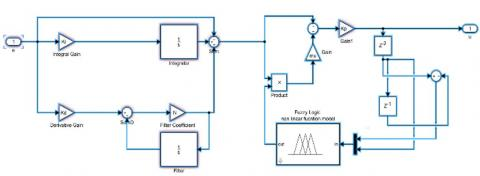Figure 1. Matlab/Simulink model of the LQR-based fuzzy-immune PID structure

5. Application

In this section, in order to validate the proposed PID controller structure, we apply it for a networked (distant) control of a greenhouse temperature.

5.1 System presentation

Greenhouse constructions are designed all over the world for extending the production season of plants and different crops in a suitable climate. Therefore, it is worth to improve the environmental conditions and overcome the external influences to guarantee plantations with high quality, low cost and energy consumption. The main parameters that should be managed in a greenhouse are: air temperature, air moisture, CO2 concentration, light density. This is why the greenhouse is equipped by several controllers, sensors and actuators .

In this paper, we will use an experimental model describing the dynamics of the greenhouse climate system (especially the temperature inside), it is represented by a parametric ARX model as follow :

$A\left( {{q}^{-1}} \right)y\left( t \right)=B\left( {{q}^{-1}} \right)u\left( t-nk \right)+e\left( t \right)$          (15)

with:

$\ A\left( {{q}^{-1}} \right)=1+{{a}_{1}}{{q}^{-1}}+{{a}_{2}}{{q}^{-2}}+...{{a}_{na}}{{q}^{-na}}$.

$\ B\left( {{q}^{-1}} \right)={{b}_{0}}+{{b}_{1}}{{q}^{-1}}+{{b}_{2}}{{q}^{-2}}+...{{a}_{nb}}{{q}^{-nb}}$.

and, A(q-1), B(q-1) represent the nominal dynamic of the system, u(t-nk) is the input signal, y(t) is the output signal, nk is the number of input samples occurred before the input affects the output, e(t) is the disturbance signal, q-1 is the delay operator, θ=[a1 a2 ... ana b1 b2 ... bnb]is the vector of the estimated parameters.

Using experimental data and MATLAB identification toolbox, the following temperature control discrete transfer function are obtained :

$H\left( {{q}^{-1}} \right)=\frac{{{r}_{1}}{{q}^{-1}}+{{r}_{2}}{{q}^{-2}}}{1-{{l}_{1}}{{q}^{-1}}+{{l}_{2}}{{q}^{-2}}}=\frac{0.2215{{q}^{-1}}+0.2215{{q}^{-2}}}{1-1.384{{q}^{-1}}+0.3989{{q}^{-2}}}$           (16)

Or in the frequency (Laplace) domain:

$H\left( s \right)=\frac{{{b}_{0}}s+{{b}_{1}}}{{{a}_{0}}{{s}^{2}}+{{a}_{1}}s+{{a}_{2}}}=\frac{-0.5149s+67.9}{{{s}^{2}}+9.205s+2.192}$              (17)

5.2 Networked control of the greenhouse temperature

## 2.jpg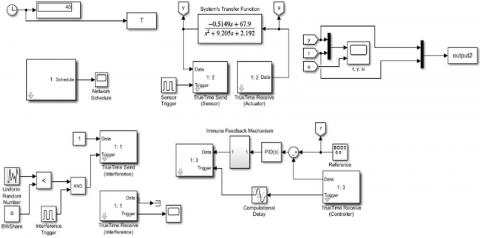Figure 2. Matlab/Simulink model of the networked greenhouse temperature control system

Networked control systems in which the control loop signals transits over a network medium have known a great interest these last years, this is due to the communication channels developments, the reduced installation costs and the easier maintainability. On the other hand, these systems present some uncertainties (transmission delays, packet loss… etc.) that affect the control loop performances, this is why it is mandatory to adopt a robust control method to handle these difficulties. In the following, we present the system on which we will apply the proposed controller structure, it concerns a greenhouse temperature networked control system based on the model presented in the previous section. Ethernet is chosen as a communication channel, it is considered as the most practical network solution to control distant systems thanks to its easy configuration, low cost and higher communication rates [10, 11]. In addition, we used three nodes (controller, network and sensors) with the following Ethernet network parameters (Table 1).

Table 1. Ethernet parameters

 Parameters values Number of nodes Data rate Minimum frame size 3 80000 bits/s 80

Figure 2 presents the SIMULINK model of the networked greenhouse temperature control system described below, here the Ethernet network is simulated by TrueTime software, this is the most used network simulator thanks to its rich network components library (for more details on this software see .

In the following sections, three controller structures will be applied to control the networked greenhouse temperature system model: the classical PID, the fuzzy-immune PID and the proposed LQR-based fuzzy-immune PID. The results in terms of time performances and robustness margins will be presented, commented and compared. Figure 2 represents the Simulink/Truetime model of the greenhouse temperature networked control system. Three nodes compose it: the system transfer function, the controller and the perturbation (interferences). Note that the subsystem representing the immune feedback mechanisms is integrated into the control loop in the second and the third application only.

5.3 Greenhouse temperature networked control using an optimally tuned classical PID

In the first application, our system is controlled by the classical PID structure, the main control goal here is to minimize the tracking error in a minimum time, for that, the PID parameters Kp, Ki and Kd are optimally adjusted by minimizing the Integral Time Absolute Error (ITAE) criterion, it is mathematically expressed by :

$J=\int\limits_{0}^{\infty }{t\left| e\left( t \right) \right|}dt$             (18)

Then, the PID parameters tuning optimization problem is expressed as follow:

$MI{{N}_{{{K}_{p}},{{K}_{i}},{{K}_{d}}}}\int\limits_{0}^{\infty }{t\left| e\left( t \right) \right|}dt$              (19)

This optimization problem can be solved by many software, in our case we used the optimization toolbox of MATLAB, and we obtained the following optimal PID parameters: Kp=0.3893, Ki=-0.0022, Kd=0.0007.

5.4 Greenhouse temperature networked control using the fuzzy-immune PID structure

In the second application, we used the fuzzy-immune PID controller structure with η=10 (the best value in terms of time performances and robustness after several tests), here the PID parameters are also optimally tuned by resolving equation (19) using the same software as in the previous application, we obtained the following values: Kp=0.4371,  Ki=0.3107,  Kd=0.0779.

5.5 Greenhouse temperature networked control using the proposed LQR-based fuzzy-immune PID

For the third application, we used the proposed LQR-based fuzzy-immune PID controller, it uses the same structure as the fuzzy-immune one, but the parameters of the PID are adjusted using the algorithm described in section 3, with the following matrix: Q=0.06*I (I is the identity matrix) and R=0.7.

The LQR-based fuzzy-immune PID optimal parameters values are founded using the same software as in the previous applications as follow: Kp=0.2812, Ki=0.2881, Kd=0.0310.

6. Simulation, Results and Discussion

In order to determine the time performances and the robustness of the above control methods, we tested them according to different network disturbances scenarios representing variations in the rate of packets loss probability and the bandwidth share BW, respectively:

Case (1): Packets loss probability=0, BW share=0.

Case (2): Packets loss probability=0, BW share=0.3.

Case (3): Packets loss probability=0, BW share=0.5.

Case (4): Packets loss probability=0.3, BW share=0.

Case (5): Packets loss probability=0.4, BW share=0.

Case (6): Packets loss probability=0.3, BW share=0.3.

Case (7): Packets loss probability=0.5, BW share=0.5.

Figures 3 to 9 represents a square signal tracking of the greenhouse temperature networked control model with the three approaches, in the seventh perturbation scenarios described in the above section. One can notice the following:

## 3.jpgFigure 3. Square signal tracking Case (1)

## 4.jpgFigure 4. Square signal tracking Case (2)

## 5.jpg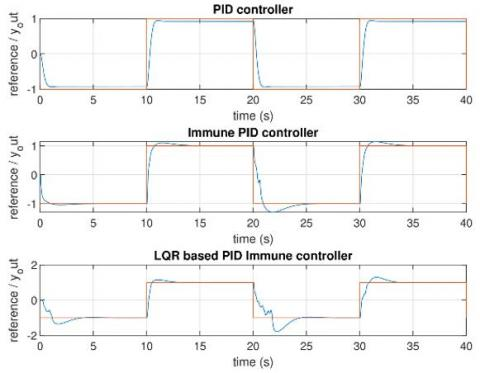Figure 5. Square signal tracking Case (3)

## 6.jpgFigure 6. Square signal tracking Case (4)

## 7.jpgFigure 7. Square signal tracking Case (5)

## 8.jpg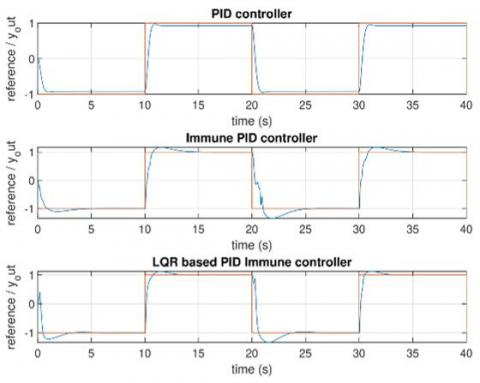Figure 8. Square signal tracking Case (6)

## 9.jpg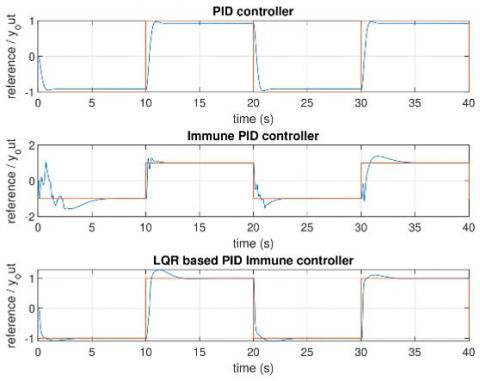Figure 9. Square signal tracking Case (7)

The optimal PID controller stills robust but a static error is maintained in all scenarios.

The fuzzy-immune PID controller gives good time performances except in the case of high packets loss percentage, but we notice a very important overshoot.

The proposed LQR-based fuzzy-immune PID controller conserves good time performances despite all the network uncertainties.

6.1 Robustness margins

The time performances were evaluated in the previous section, still the robustness margins, for that, we calculate the most used robustness margins: the gain margins Mg, the phase margins ϕg and the delay margin Δd. The results are summarized by the following Table 2:

Table 2. Robustness margins

 Controllers/margins Mg (dB) ϕg (deg) Δd Optimal PID 52.0685 76.7782 0.4775 Fuzzy-immune PID 81.8488 59.1217 2390.2 LQR-based fuzzy-immune PID 81.8502 49.6752 1377.8

One can notice the following:

The optimal classical PID controller gives the highest phase margin and a good gain margin, but also too short delay margin which refers to the delay amount added to the open loop system before it becomes unstable.

The fuzzy-immune PID and the LQR-based fuzzy-immune PID controllers have a nearby gain robustness, with slightly larger values for the second one.

From all these results, we can say that the LQR-based fuzzy-immune PID controller is the most robust and have the best time performances comparatively with the other tested controllers.

7. Conclusions

A novel LQR based fuzzy immune PID controller inspired from the biological immune system is introduced in this paper. It is modelled using fuzzy sets and its parameters are tuned using the robust LQR based algorithm. The application of the proposed controller for a networked greenhouse temperature control system shows good time performances and high robustness against several network uncertainties. The comparison of the obtained results with those of other PID controller structures, respectively, classical and fuzzy-immune ones confirms the superiority of the proposed approach in terms of time performances and robustness against the network uncertainties. On the other hand, fuzzy modeling of non-linear immune function, which is the key to the success of the proposed method, is still insufficient to express its dynamic behavior precisely. Further methods will be studied in future works.

References

 Borase, R.P., Maghade, D.K., Sondkar, S.Y., Pawar, S.N. (2021). A review of PID control, tuning methods and applications. International Journal of Dynamics and Control, 9(2): 818-827. https://doi.org/10.1007/s40435-020-00665-4

 Domingues, P.H., Freire, R.Z., Coelho, L.D.S., Ayala, H.V. (2019). Bio-inspired multiojective tuning of PID-controlled antilock braking systems. In 2019 IEEE Congress on Evolutionary Computation (CEC), pp. 888-895. https://doi.org/10.1109/CEC.2019.8790023

 Li, J., Gómez-Espinosa, A. (2018). Improving PID control based on Neural Network. In 2018 International Conference on Mechatronics, Electronics and Automotive Engineering (ICMEAE), pp. 186-191. https://doi.org/10.1109/ICMEAE.2018.00042

 Marino, A., Neri, F. (2019). PID tuning with neural networks. In Asian Conference on Intelligent Information and Database Systems, pp. 476-487. https://doi.org/10.1007/978-3-030-14799-0_41

 Singh, R., Bhushan, B. (2019). Adaptive neuro-fuzzy-PID and fuzzy-PID-based controller design for helicopter system. In Applications of Computing, Automation and Wireless Systems in Electrical Engineering, pp. 281-293. https://doi.org/10.1007/978-981-13-6772-4_25

 Jayachitra, A., Vinodha, R. (2014). Genetic algorithm based PID controller tuning approach for continuous stirred tank reactor. Advances in Artificial Intelligence, 2014: 791230. https://doi.org/10.1155/2014/791230

 Takahashi, K., Yamada, T. (1998). Application of an immune feedback mechanism to control systems. JSME International Journal Series C Mechanical Systems, Machine Elements and Manufacturing, 41(2): 184-191. https://doi.org/10.1299/jsmec.41.184

 Yuan, G.L., Liu, J.Z., Tan, W., Liu, X.J. (2007). The research and application of immune feedback control in the load control system of tube mill. In 2007 Chinese Control Conference, pp. 350-353. https://doi.org/10.1109/CHICC.2006.4347121

 Li, Z. (2010). Intelligent fuzzy immune PID controller design for multivariable process control system. In 2010 8th World Congress on Intelligent Control and Automation, pp. 4953-4957. https://doi.org/10.1109/WCICA.2010.5554805

 Bouchebbat, R., Gherbi, S. (2017). Design and application of fuzzy immune PID adaptive control based on particle swarm optimization in thermal power plants. In 2017 6th International Conference on Systems and Control (ICSC), pp. 33-38. https://doi.org/10.1109/ICoSC.2017.7958656

 Tang, B., Wang, J., Zhang, Y. (2015). A delay-distribution approach to stabilization of networked control systems. IEEE Transactions on Control of Network Systems, 2(4): 382-392. https://doi.org/10.1109/TCNS.2015.2426731

 Rouamel, M., Gherbi, S., Bourahala, F. (2020). Robust stability and stabilization of networked control systems with stochastic time-varying network-induced delays. Transactions of the Institute of Measurement and Control, 42(10): 1782-1796. https://doi.org/10.1177/0142331219895931

 Tang, Y., Du, F., Cui, Y., Zhang, Y. (2018). New smith predictive fuzzy immune PID control algorithm for MIMO networked control systems. EURASIP Journal on Wireless Communications and Networking, 2018(1): 1-15. https://doi.org/10.1186/s13638-018-1229-8

 Wang, P., Hao, M., Ji, Q. Z. (2014). Study on fuzzy immune PID controller for main steam pressure control system in marine steam power plant. In Applied Mechanics and Materials, 686: 89-94. https://doi.org/10.4028/www.scientific.net/amm.686.89

 Shojaei Barjuei, E., Ortiz, J. (2021). A comprehensive performance comparison of linear quadratic regulator (LQR) controller, model predictive controller (MPC), H∞ loop shaping and μ-synthesis on spatial compliant link-manipulators. International Journal of Dynamics and Control, 9(1): 121-140. https://doi.org/10.1007/s40435-020-00640-z

 Mukhopadhyay, S. (1978). PID equivalent of optimal regulator. Electronics Letters, 14(25): 821-822. https://doi.org/10.1049/el: 19780555

 Fitz-Rodríguez, E., Kubota, C., Giacomelli, G.A., Tignor, M.E., Wilson, S.B., McMahon, M. (2010). Dynamic modeling and simulation of greenhouse environments under several scenarios: A web-based application. Computers and Electronics in Agriculture, 70(1): 105-116. https://doi.org/10.1016/j.compag.2009.09.010

 Cojuhari, I., Izvoreanu, B., Moraru, D., Speian, A., Romanov, A. (2012). Greenhouse temperature control system. 11th International Conference on Development and Application Systems, Suceava, Romania, pp. 33-36.

 Martin, O., Dan, H., Anton, C. (2010). TrueTime 2.0 bêta. Reference Manual. Lund Institute of Technology. Sweden.

 Maiti, D., Acharya, A., Chakraborty, M., Konar, A., Janarthanan, R. (2008). Tuning PID and PI/λDδ controllers using the integral time absolute error criterion. In 2008 4th International Conference on Information and Automation for Sustainability, pp. 457-462. https://doi.org/10.1109/ICIAFS.2008.4783932